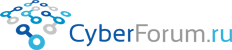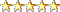Наши страницы
8Observer8
 Войти Регистрация Восстановить пароль
 Тесты Блоги Социальные группы Поиск Сообщения за день Все разделы прочитаны# Solving problems (tasks) with TDD in Python

Запись от 8Observer8 размещена 10.01.2019 в 20:14
Обновил(-а) 8Observer8 10.01.2019 в 20:15

This is a problem: Карта сокровищ

When I start solve these tasks I write a unit test, like this:

test_treasure.py

Python
 ```1 2 3 4 5 6 7 8 9 10 11 12 13 14 15 ``` ```import unittest   class TestTreasure(unittest.TestCase):       def test_one_treasure(self):         # Arrange         treasure = Treasure()         input = [(15, 16)]         expectedAmount = 1           # Act         actualAmount = treasure.GetAmount(input)           # Assert         self.assertEqual(expectedAmount, actualAmount)```
I run it using command:

Цитата:
python -m unittest
I see the error message in the terminal:

Цитата:
treasure = Treasure()
NameError: name 'Treasure' is not defined
I define the Treasure class:

treasure.py

Python
 ```1 2 3 ``` ```class Treasure:     def GetAmount(self, input):         return -1```
And import it to test_treasure.py

Python
 ```1 2 3 ``` ```import unittest   from treasure import Treasure```
Run test:
Цитата:
python -m unittest
I see:

Python
 ```1 2 ``` ```    self.assertEqual(expectedAmount, actualAmount) AssertionError: 1 != -1```
I start to implement a logic to make my first test pass. If you want you can to write the logic and add second test.

You can make the test pass like this:

treasure.py

Python
 ```1 2 3 4 5 ``` ```class Treasure:     def GetAmount(self, input):         if len(input) == 1:             return 1         return -1```
I run test and see that test is pass:
Цитата:
> python -m unittest
.
----------------------------------------------------------------------
Ran 1 test in 0.001s

OK
Add the second test with two treasures. Expected amount is equal to 2 in the second test:

test_treasure.py:

Python
 ```1 2 3 4 5 6 7 8 9 10 11 12 13 14 15 16 17 18 19 20 21 22 23 24 25 26 27 28 29 ``` ```import unittest   from treasure import Treasure   class TestTreasure(unittest.TestCase):       def test_one_treasure(self):         # Arrange         treasure = Treasure()         input = [(15, 16)]         expectedAmount = 1           # Act         actualAmount = treasure.GetAmount(input)           # Assert         self.assertEqual(expectedAmount, actualAmount)       def test_two_treasures(self):         # Arrange         treasure = Treasure()         input = [(15, 16), (16, 18)]         expectedAmount = 2           # Act         actualAmount = treasure.GetAmount(input)           # Assert         self.assertEqual(expectedAmount, actualAmount)```
Run tests:

Цитата:
python -m unittest
See the result:

Python
 ```1 2 3 ``` ```test_treasure.py", line 29, in test_two_treasures    self.assertEqual(expectedAmount, actualAmount) AssertionError: 2 != -1```
We need to pass test again. This is a process of TDD (Test-Driven Development)
Размещено в Без категории
Просмотров 226 Комментарии 0
Всего комментариев 0
Комментарии
 КиберФорум - форум программистов, компьютерный форум, программирование Реклама - Обратная связь